×

Search anything:

# Activation Functions in Machine Learning: A Breakdown

#### Machine Learning (ML)Get this book -> Problems on Array: For Interviews and Competitive Programming

The goal of this article at OpenGenus, is to simplify Math-heavy and counter-intuitive topic of Activation Functions in Machine Learning that can trip up newcomers to this exciting field!

We have covered the basics of Activation functions intuitively, its significance/ importance and its different types like Sigmoid Function, tanh Function and ReLU function.

## A Brief Intro to Neural Neworks

The functions we shall be talking about are used often in the creation of Artificial Neural Networks (ANNs), which are used in Deep Learning - a subdomain of Machine Learning.

The difference between the two is aptly stated by Bernard Marr:

Instead of teaching computers to process and learn from data (which is how machine learning works), with deep learning, the computer trains itself to process and learn from data.

A big advantage of deep learning models is that they do not necessarily need structured/labeled data. They can be used for classification and regression tasks, and also for reinforcement learning. An amazing visual introduction to the field is the video by Grant Sanderson, aka 3Blue1Brown on YouTube. The video explains how neural networks function and can classify handwritten digits.

Taking inspiration from the human brain, the concept of a neural network can be traced back all the way to 1944! Billions of neurons like the one shown in the image below exist in the brain (around 86 billion, actually). A neuron is an electrically excitable cell that takes up, processes and transmits information through electrical and chemical signals. They are the basic elements of the nervous system.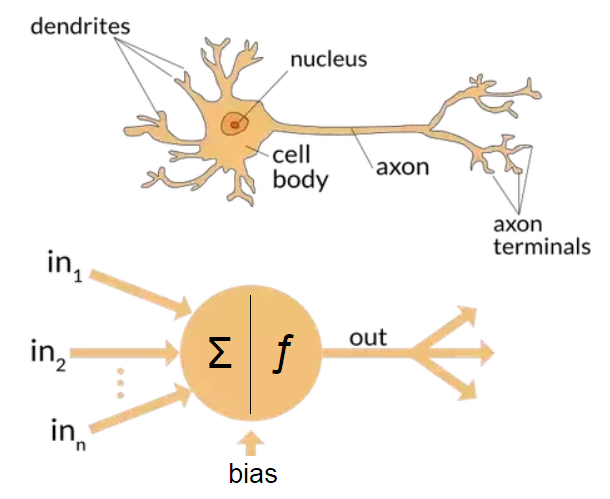An artificial neuron is shown below the biological one in the image. These are also called nodes and are often represented as circles in ANN diagrams. Each connection can transmit a signal to other neurons, like the synapses in the brain do by firing. An artificial neuron that receives a signal then processes it and can signal neurons connected to it. A collection of such neurons in different layers forms an ANN.

Each node in a network receives a set of inputs, and each connection has a weight associated with it. The weighted sum of these inputs (a bias is also added to this usually) is passed on to a function that gives us a set of outputs for that neuron. This is the Activation Function. In the diagram above, the weighted sum is denoted by the sigma symbol (Σ) and the activation function by the lowercase ƒ.

## What are Activation Functions?

As one can guess from the name, it is a mathematical function. And like all such functions it will -

• take a set of inputs
• perform some operations on them
• return a set of corresponding outputs

This set of outputs lies in a range (generally 0 to 1 or -1 to 1) depending upon the function. Thus the activation function normalizes the nodes outputs to a set range.

While that explains the "function" part of activation functions, what does the "activation" part mean?

In a way, the Activation Function determines whether (or to what extent) a signal should progress further through the network to affect the ultimate outcome. If the signals passes through, the neuron has been “activated.”

The output of the activation function of one node is passed on to the next node layer, where the same process can continue. The output received from the final layer (the output layer) becomes the model's output.

The weights of each connection symbolize how important a neuron is to another neuron to achieve a particular final output. While the network is "learning" for a task, it updates these weights to obtain the desired final output. The bias is added to help do this. It can shift the activation function to the left or to the right, just like the intercept in the equation of a line (y= mx + c) shifts the graph up or down. In this regard, the weights and biases are like knobs that can be tuned to reach the desired final output.

## Why are they important?

The activation function you choose will affect the results and accuracy of your Machine Learning model. This is why one needs to be aware about the many different kinds of activation functions, and have the awareness to choose the right ones for the right tasks.

The biggest advantage of the activation function is that it imparts non-linearity to our models. Linear functions are (as the name applies) straight lines or planes, thus being limited in their complexity. For most kinds of complex data, such a linear function would perform poorly and the model would be weak.

These non-linear functions also should be differentiable. This is required to enable backpropagation. This why the activation functions discussed in this blog shall all be non-linear ones.

Finally, we also want our function to be computationally efficient. This is because it will be calculated across thousands or even millions of neurons for each data sample.

## Popular Activation Functions

The three traditionally most-used functions that can fit our requirements are:

1. Sigmoid Function
2. tanh Function
3. ReLU Function

In this section, we discuss these and a few other variants. The mathematical formula for each function is provided, along with the graph. Note that the range of its output can be interpreted from the y-axis.

### 1. The Sigmoid Function

#### (aka Logistic Function)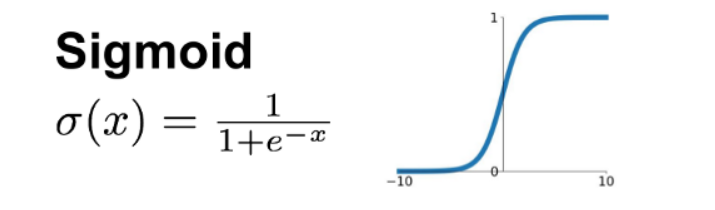• Output values bound between 0 and 1, normalizing the output of each neuron
• Clear predictions

• Vanishing gradient— for extreme values of X, there is almost no change to the prediction. This can result in the network refusing to learn further, or being too slow to reach an accurate prediction.
• Computationally expensive
• Not zero centered

### 2. The tanh Function

#### (aka Hyperbolic Tangent)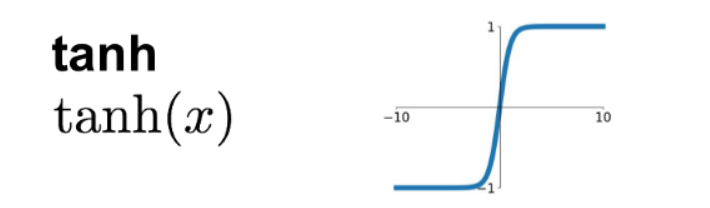• tanh will give zero-centered outputs, which is desirable

• It suffers from the same issues as the sigmoid function

### 3. The ReLU Function

#### (aka Rectified Linear Unit)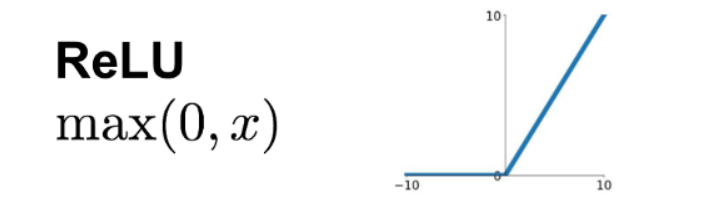• It is computationally efficient, allowing the network to converge very quickly

• "The Dying ReLU problem"— when inputs are negative (or approaching zero), the gradient of the function becomes zero. In such cases the network cannot perform backpropagation and cannot learn.

Prior to the introduction of ReLU, most neural networks used the classic sigmoid activation function or the hyperbolic tangent activation function

Nowadays, ReLU has become the default activation function of choice.

### Other Functions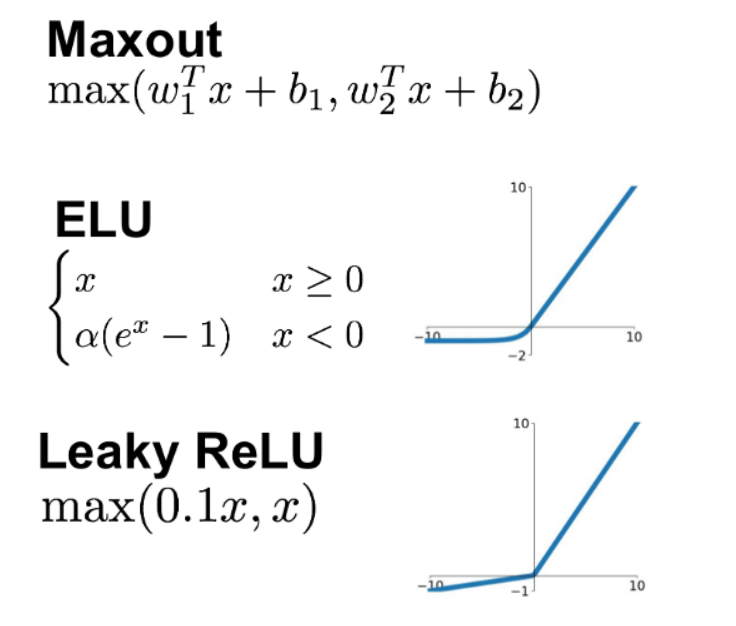There are so many more functions that are being used, and many are suited for certain types of problems rather than others. You can read about them in the ML Cheatsheet referenced below.

A newer function that has become popular is Leaky ReLU. It fixes the "Dying ReLU problem" very cleverly. This variation of ReLU has a tiny positive slope in the bottom left of the graph (so it does enable backpropagation even for negative input values). It does suffer from inconsistencies, however.

The ML Cheatsheet - Activation Functions
Types of Neural Networks
A Neural Network in 11 Lines of Code

## Test Yourself

#### What are the desirable characteristics of an Activation Function?

Normalized Output
Computational Efficiency
Non-linear and differentiable
All of these are desirable
* Computationally efficient functions help the network converge towards the final output quicker.
* Non-linear functions make it easy for the model to generalize or adapt to a variety of data, and to differentiate between outputs. The derivatives/slopes of these functions help with backpropagation.
* Normalization is needed to limit outputs; which can otherwise reach very high orders of magnitude and lead to computational issues (especially in case of very deep neural networks that have millions of parameters).

With this article at OpenGenus, you must have the complete idea of Activation function in Machine Learning. Enjoy.

#### Divij Kulshrestha

Writer and data science enthusiast!